# Kinetic Energy with Examples

KINETIC ENERGY

Objects have energy because of their motion; this energy is called kinetic energy. Kinetic energy of the objects having mass m and velocity v can be calculated with the formula given below;

Ek=1/2mv²

As you see from the formula, kinetic energy of the objects is only affected by the mass and velocity of the objects. The unit of the Ek is again from the formula kg.m²/s² or in general use joule.

Example Find the kinetic energy of the ball having mass 0,5 kg and velocity 10m/s.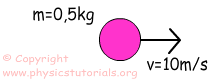Ek=1/2mv²

Ek=1/2.0, 5. (10) ²

Ek=25joule

As in the case of Kinematics we can use graphs to show the relations of the concepts here. Look at the given graph of Force vs. Distance.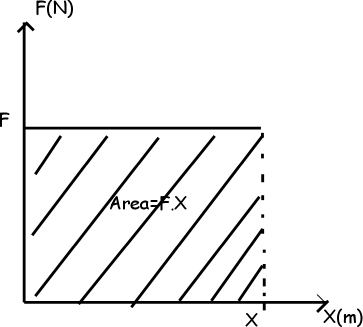Area under the force vs. distance graph gives us work

Work=Force. Distance=Area=F.X (distance)

We can find energy of the objects from their Force vs. Distance graph.

Example Find the Kinetic Energy of the object at 14m from the given graph below.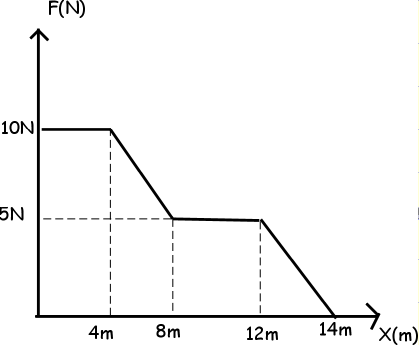We can find the total kinetic energy of the object after 14m from the graph; we use area under it to find energy.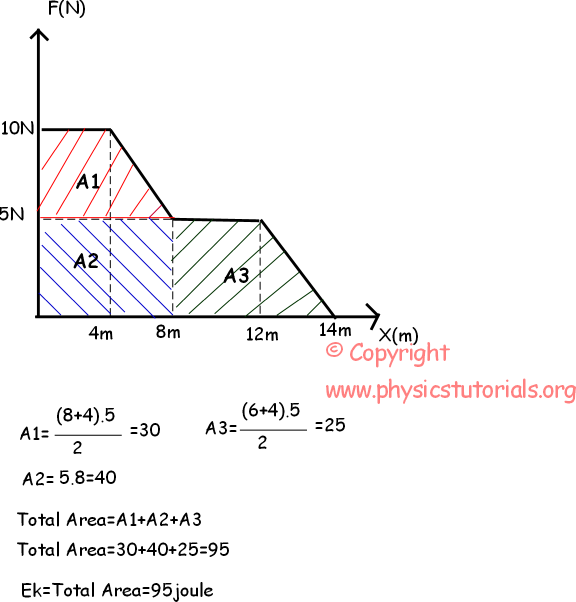Example Look at the given picture below. If the final velocity of the box is 4m/s find the work done by friction.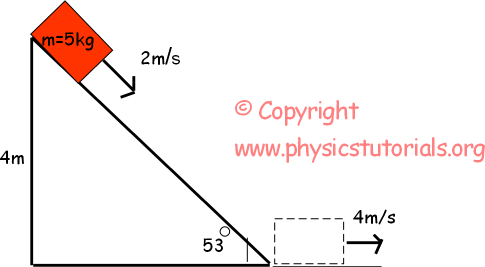At the top;

Etotal=mgh+1/2mv²

Etotal=5kg.10m/s².4m+1/2.5kg. (2m/s) ²=210joule

At the bottom;

Etotal=1/2mv²=1/2.5kg.(4m/s) ²

Etotal=40joule

The difference between the initial and final energy is used by the friction.

Work done by the friction=Efinal-Einitial=210joule-40joule=170joule

Work Power Energy Exams and Solutions

Related